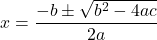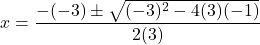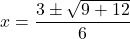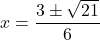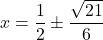## I WILL GIVE BRAINLIEST!!! Find the solutions of the quadratic equation 6x^2-6x-2=0 Choose 1 answer: (cho

Question

I WILL GIVE BRAINLIEST!!!

Find the solutions of the quadratic equation 6x^2-6x-2=0

(choice A)
A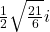(choice B)
B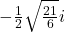(choice C)
C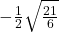(choice D)
D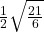in progress 0
5 months 2021-08-17T11:38:37+00:00 1 Answers 0 views 0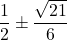Step-by-step explanation: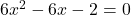Divide both sides by 2.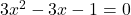We compare it to the standard form: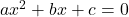We have a = 3, b = -3, c = -1.

To factor, we need two numbers that multiply to ac and add to b.

ac = 3 * (-1) = -3

-3 = -3 * 1; -3 + 1 = -2

-3 = -1 * 3; -1 + 3 = 2

There are no two integers whose product is -3 and whose sum is -3, so we cannot factor the trinomial. We use the quadratic formula to solve the equation.